# Frank Solutions for Class 10 Maths Chapter 16 Loci

Frank Solutions for Class 10 Maths Chapter 16 Loci are given here. The BYJU’S subject expert team has prepared solutions to help students obtain good marks in Maths. From the exam point of view, the solutions are solved in a simple manner, and students can secure an excellent score by solving Frank Solutions for Class 10 Maths Chapter 16. Solutions that are provided here will help you in getting acquainted with a wide variety of questions and thereby develop problem-solving skills.

Chapter 16 – Loci are a set of points with the same property. Loci can be used to construct lines and shapes accurately. These solutions are helpful for the students to cross-check with the exercise answers in the chapter to improve their solving methods.

## Download the PDF of Frank Solutions for Class 10 Maths Chapter 16 Loci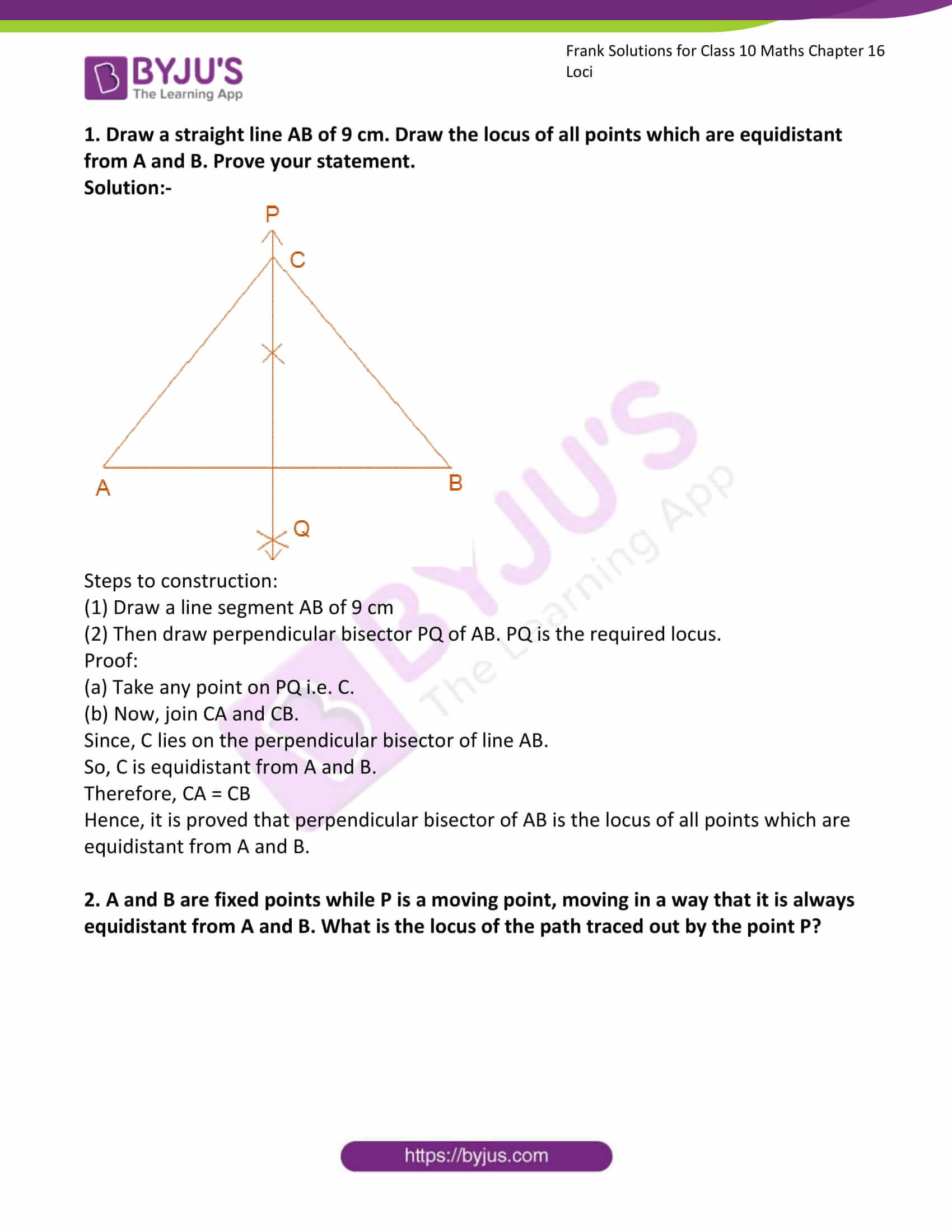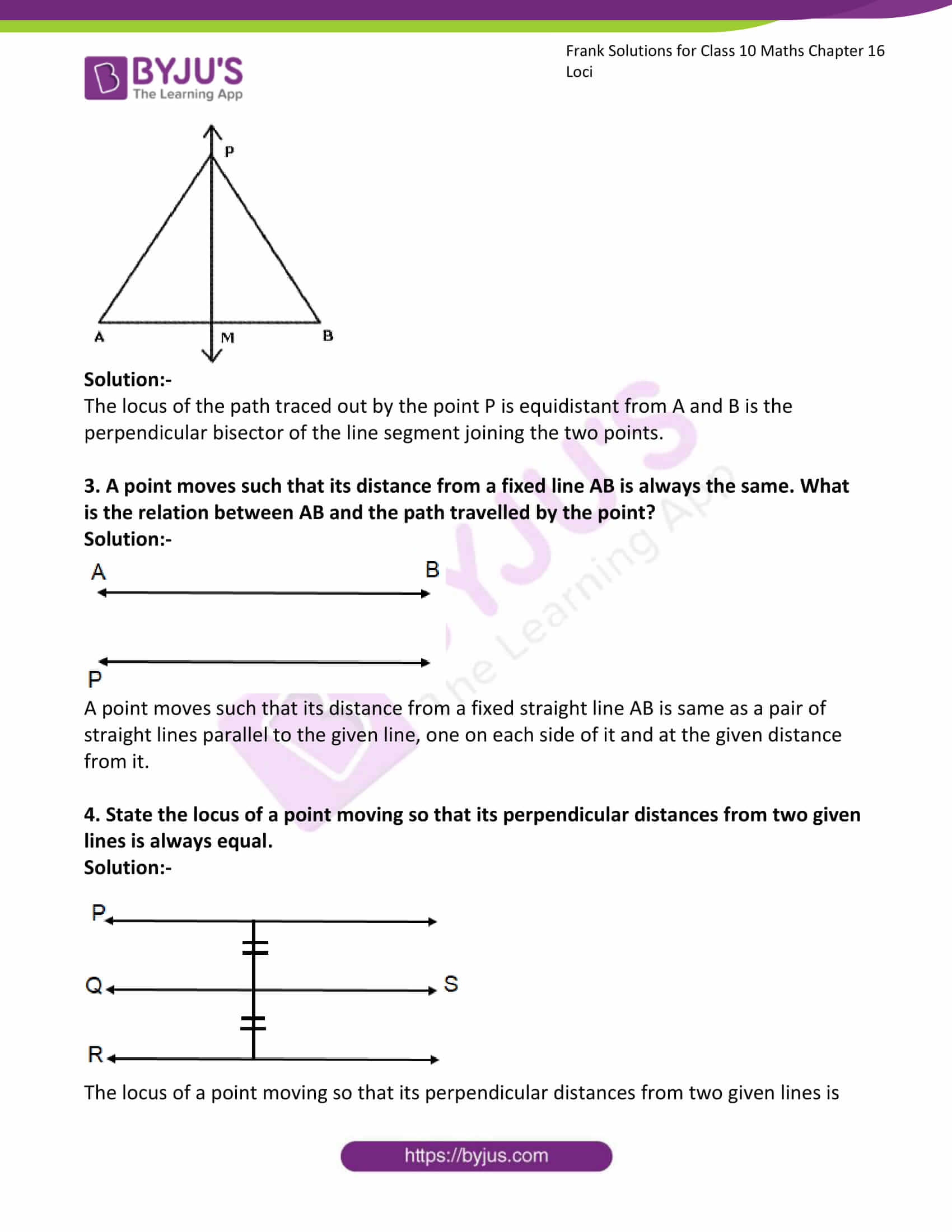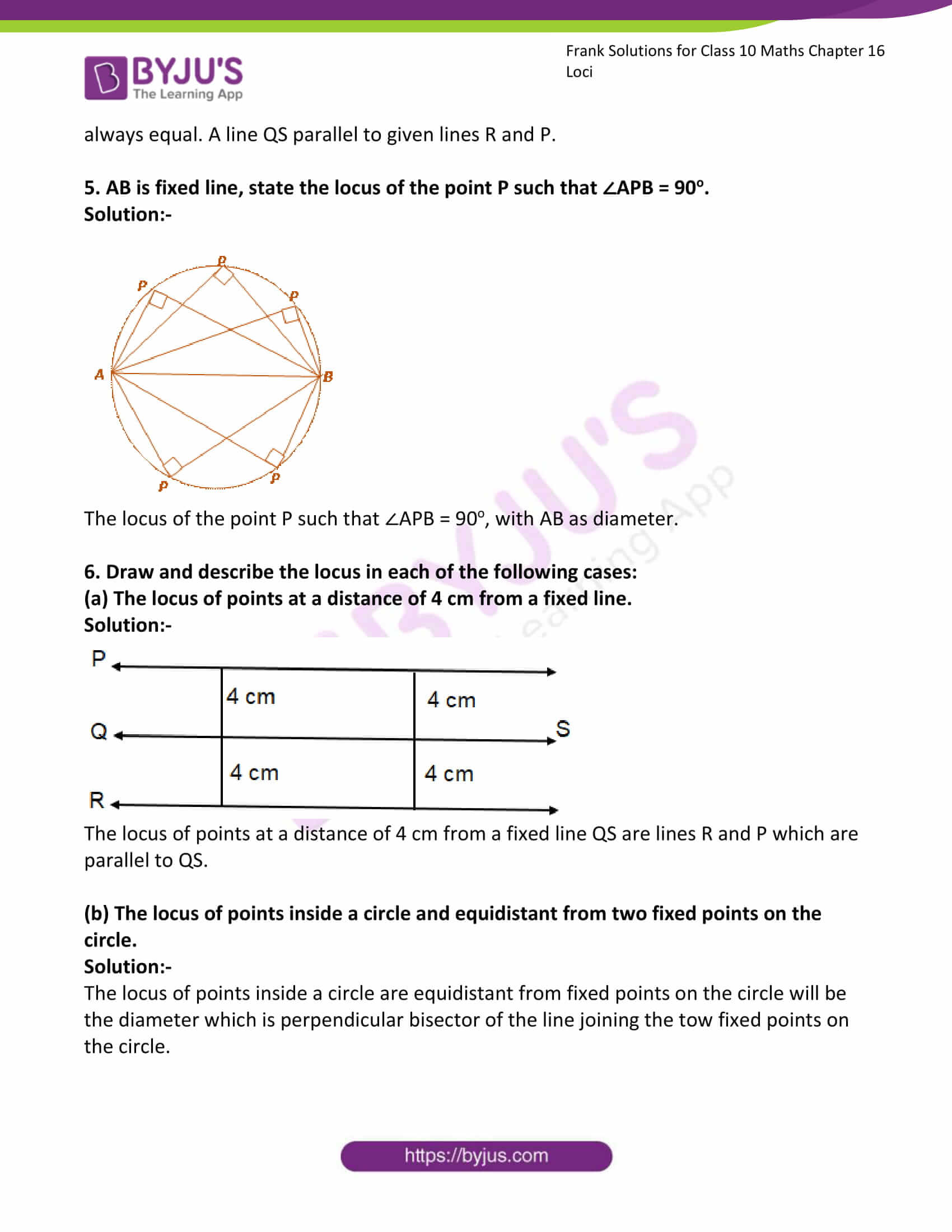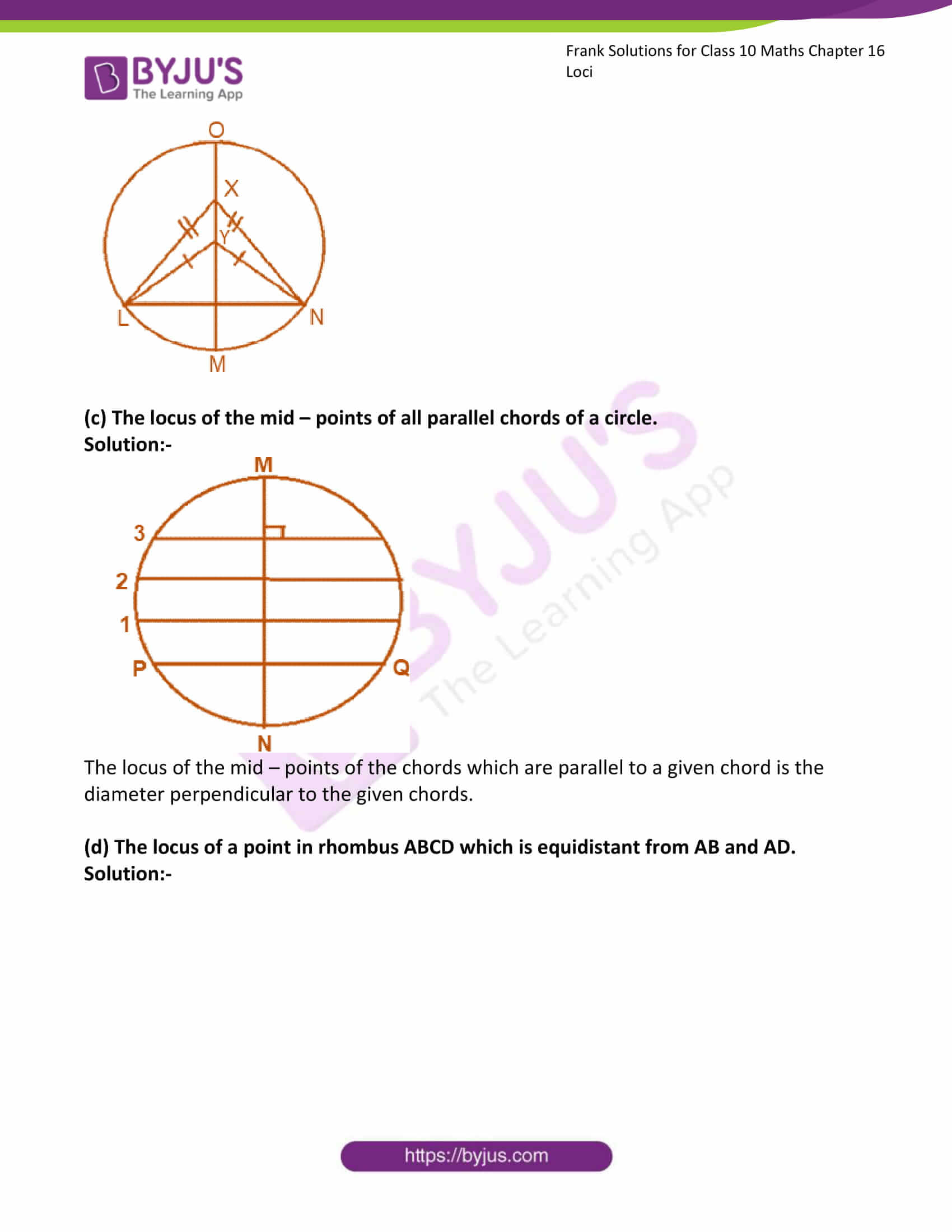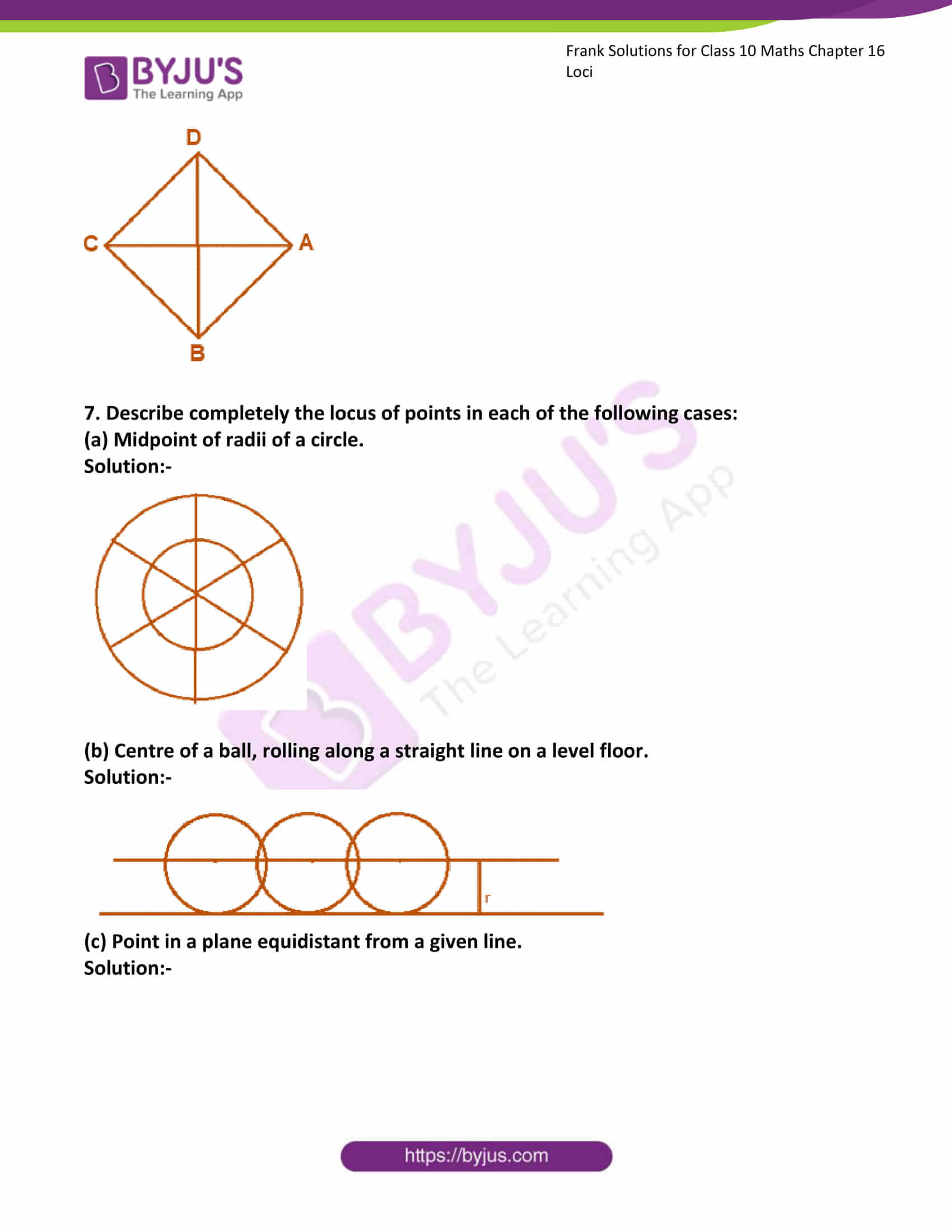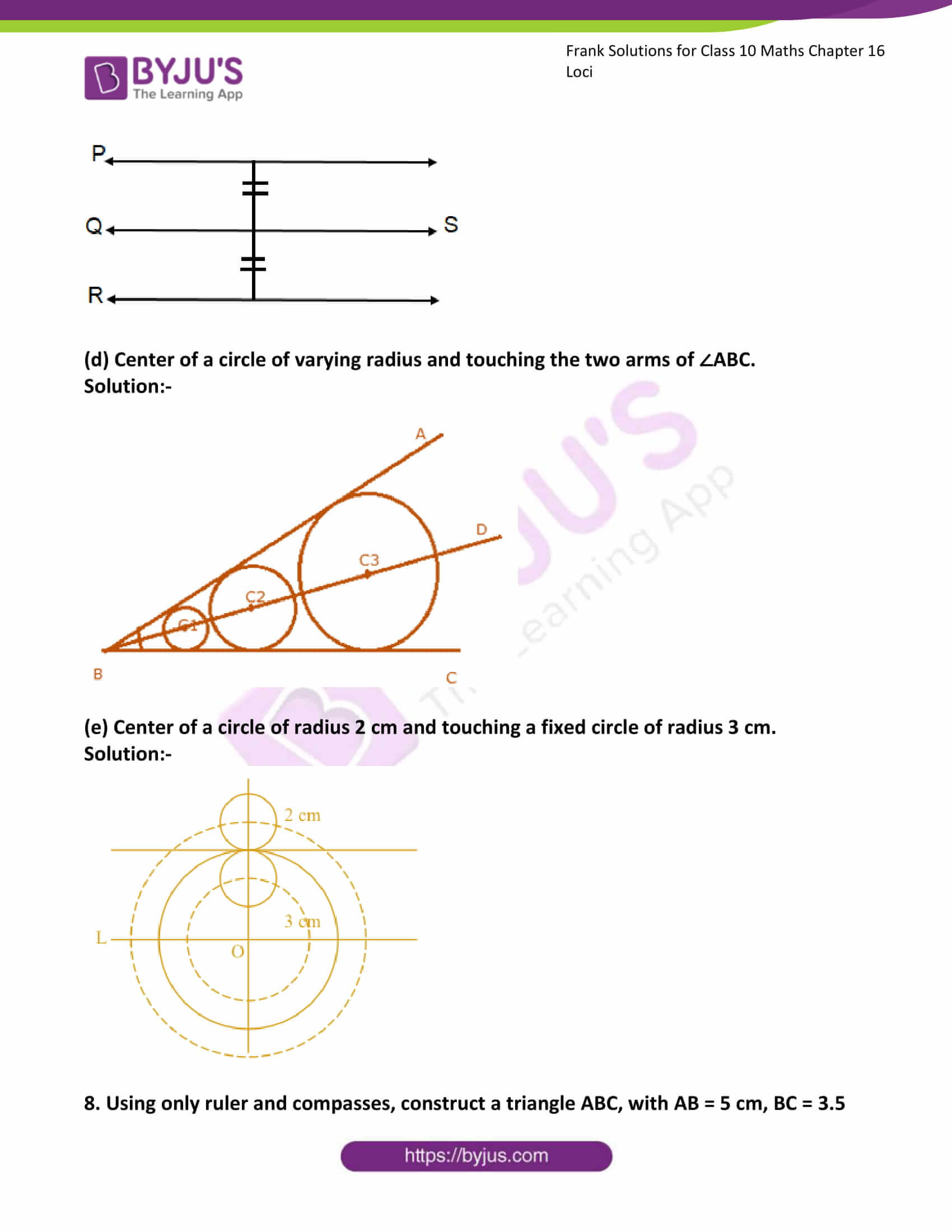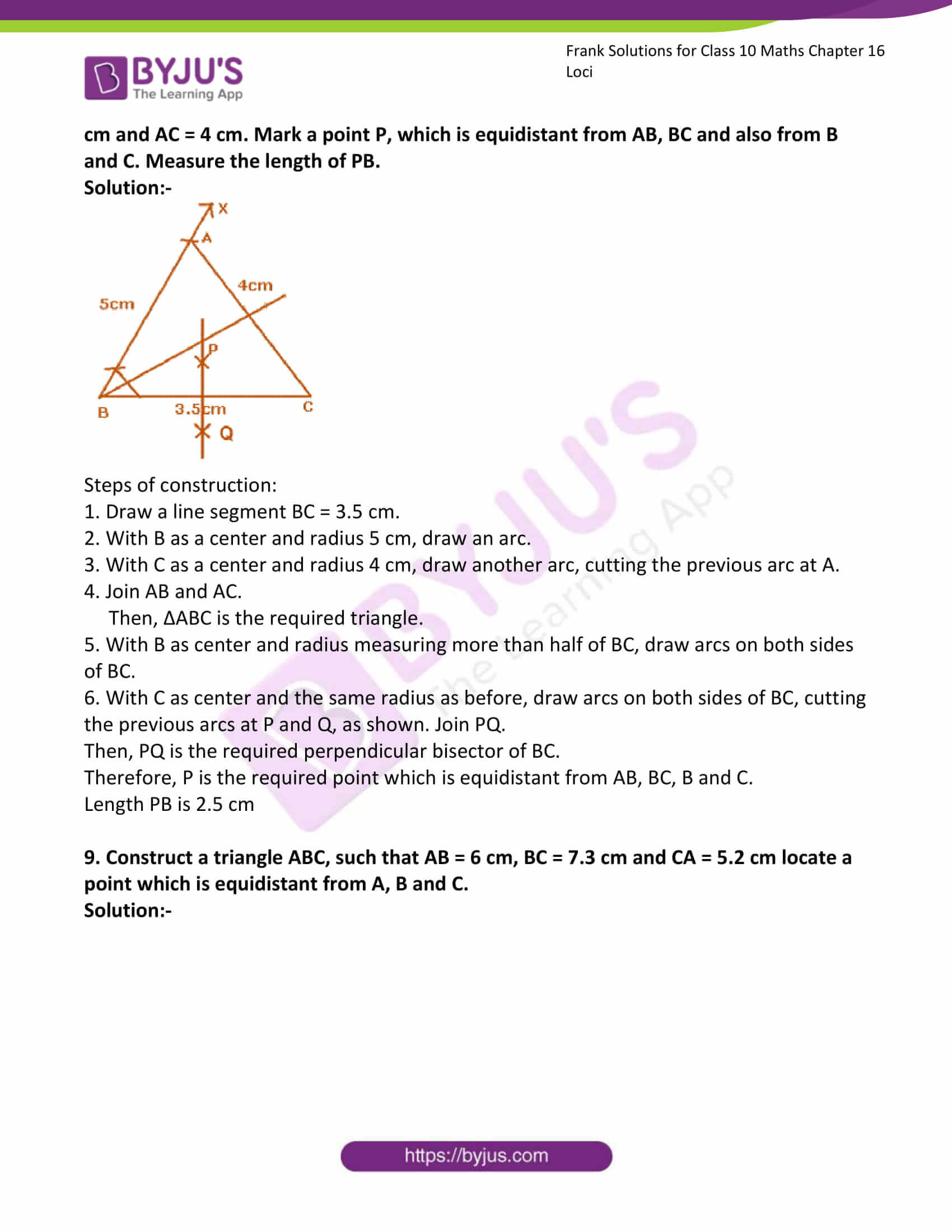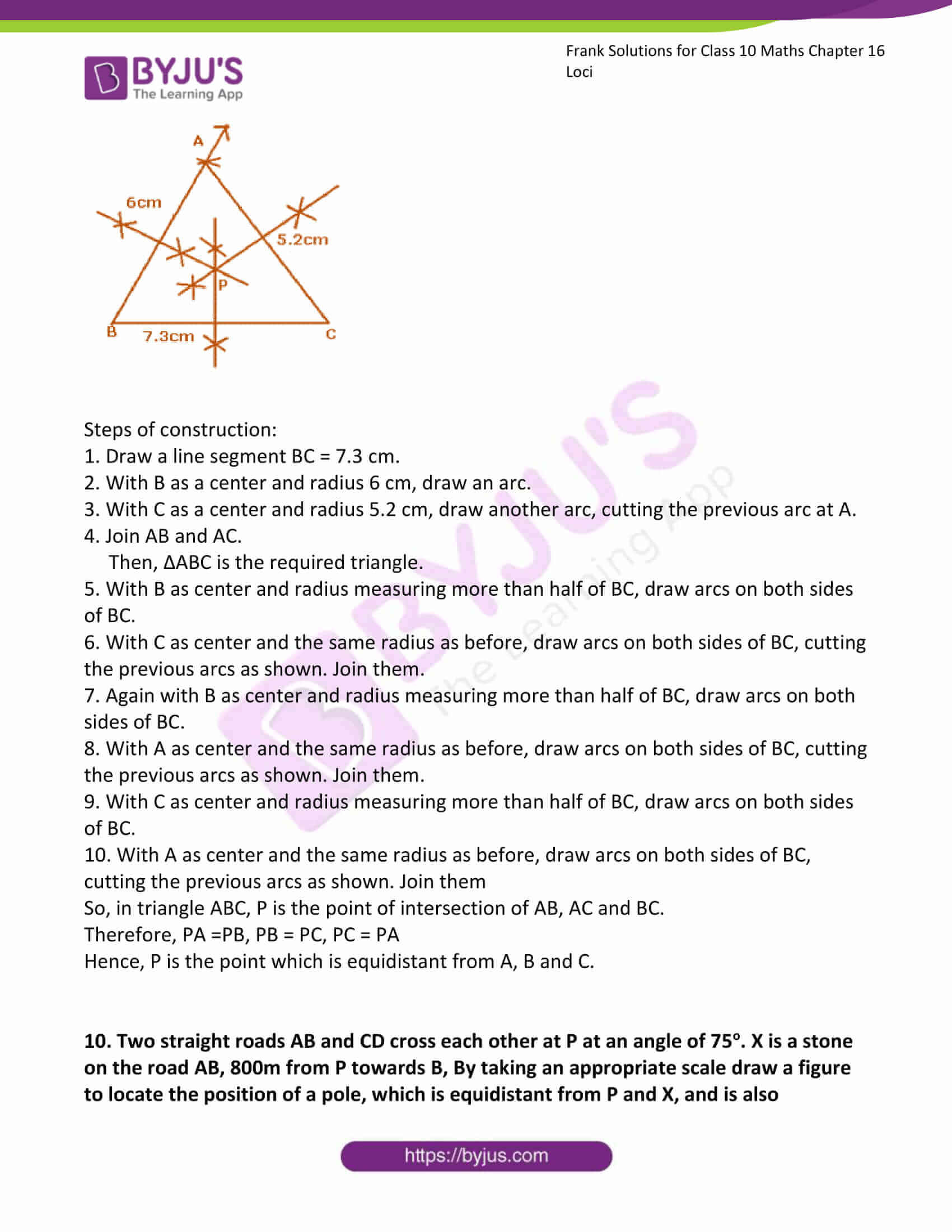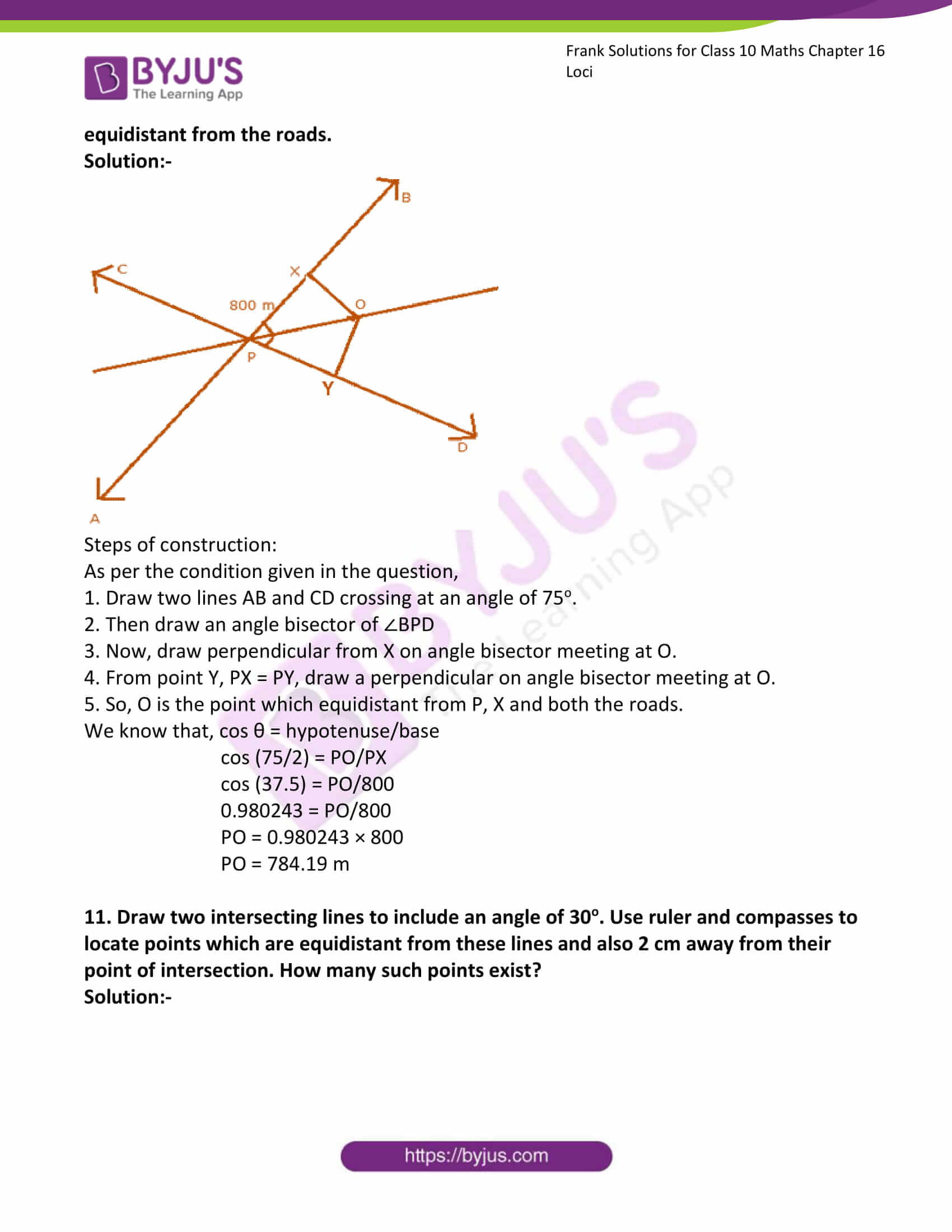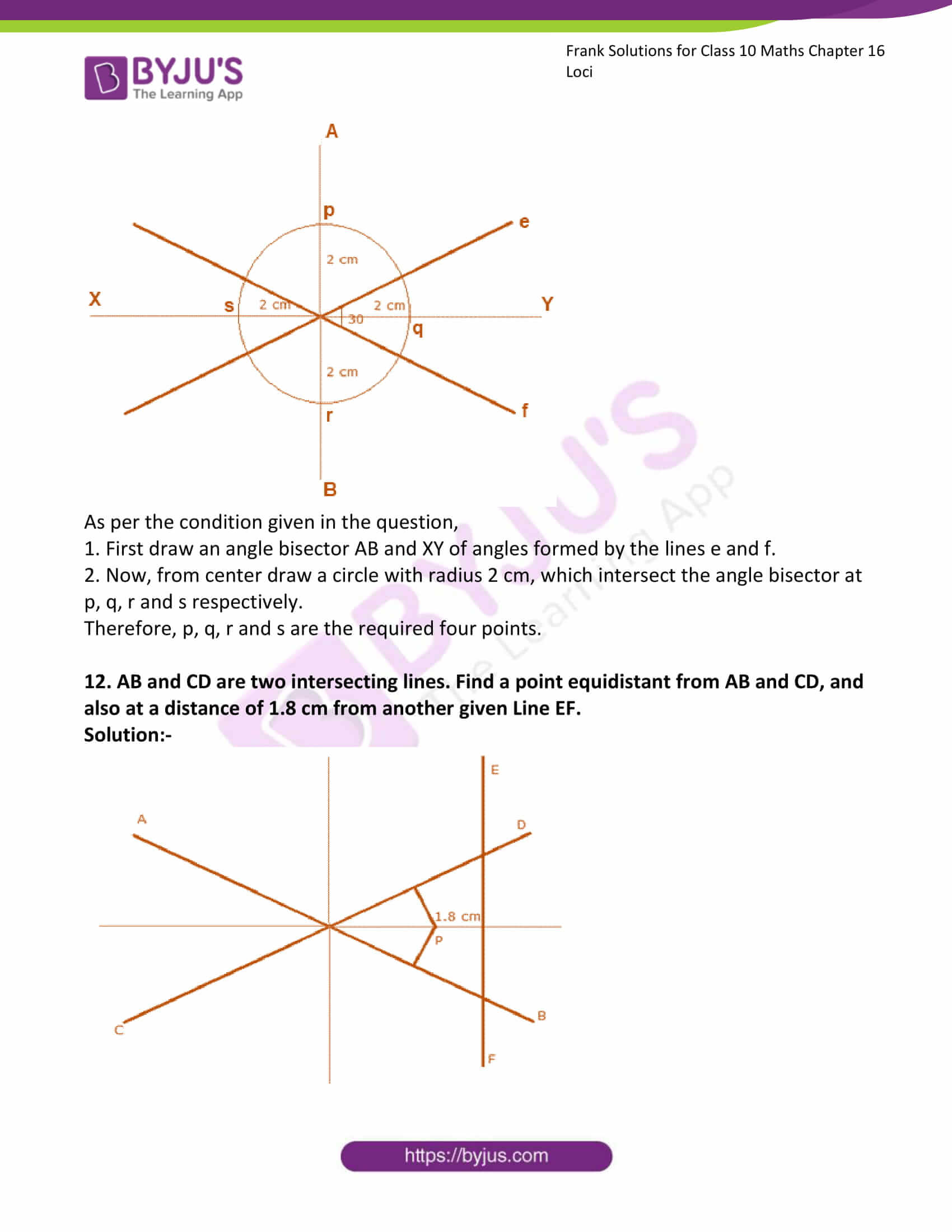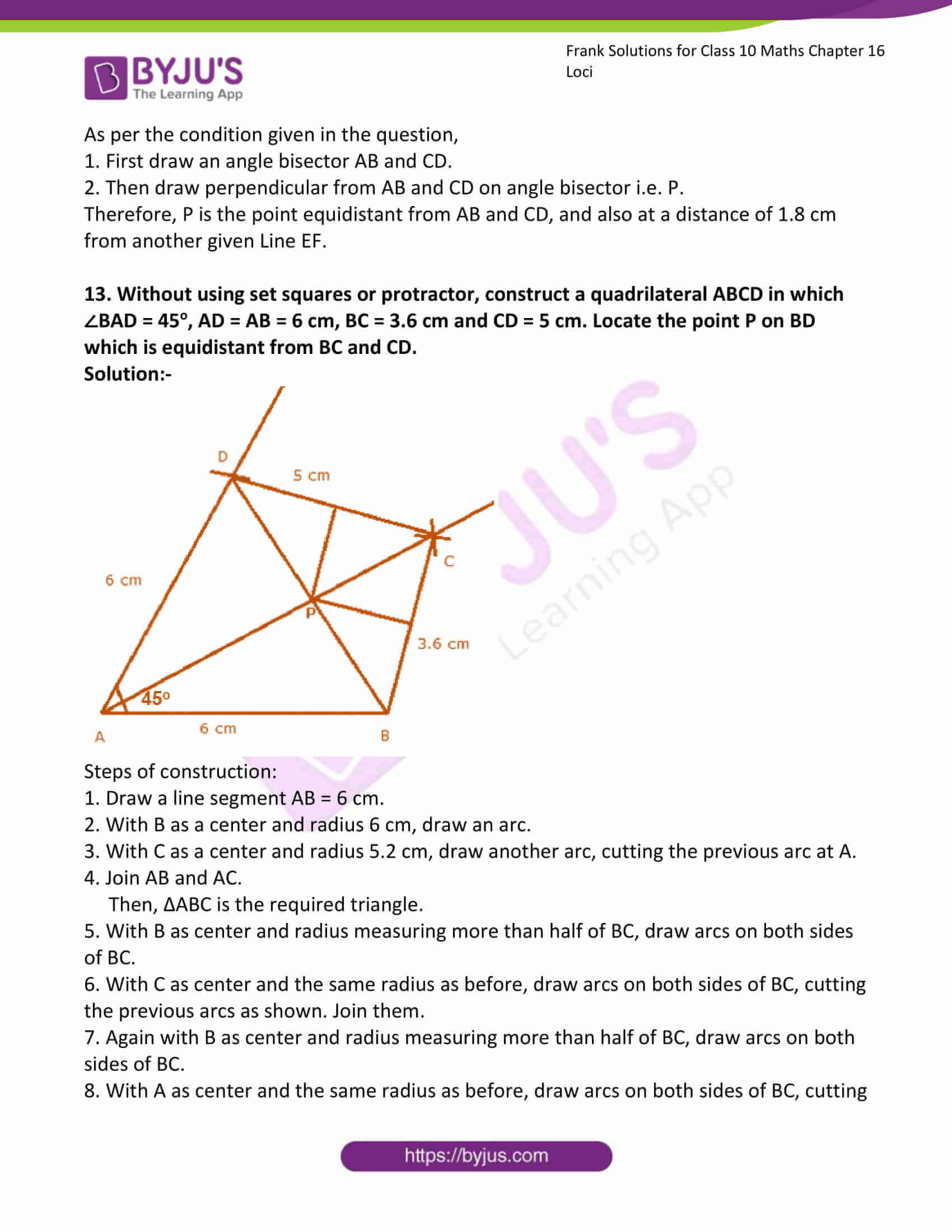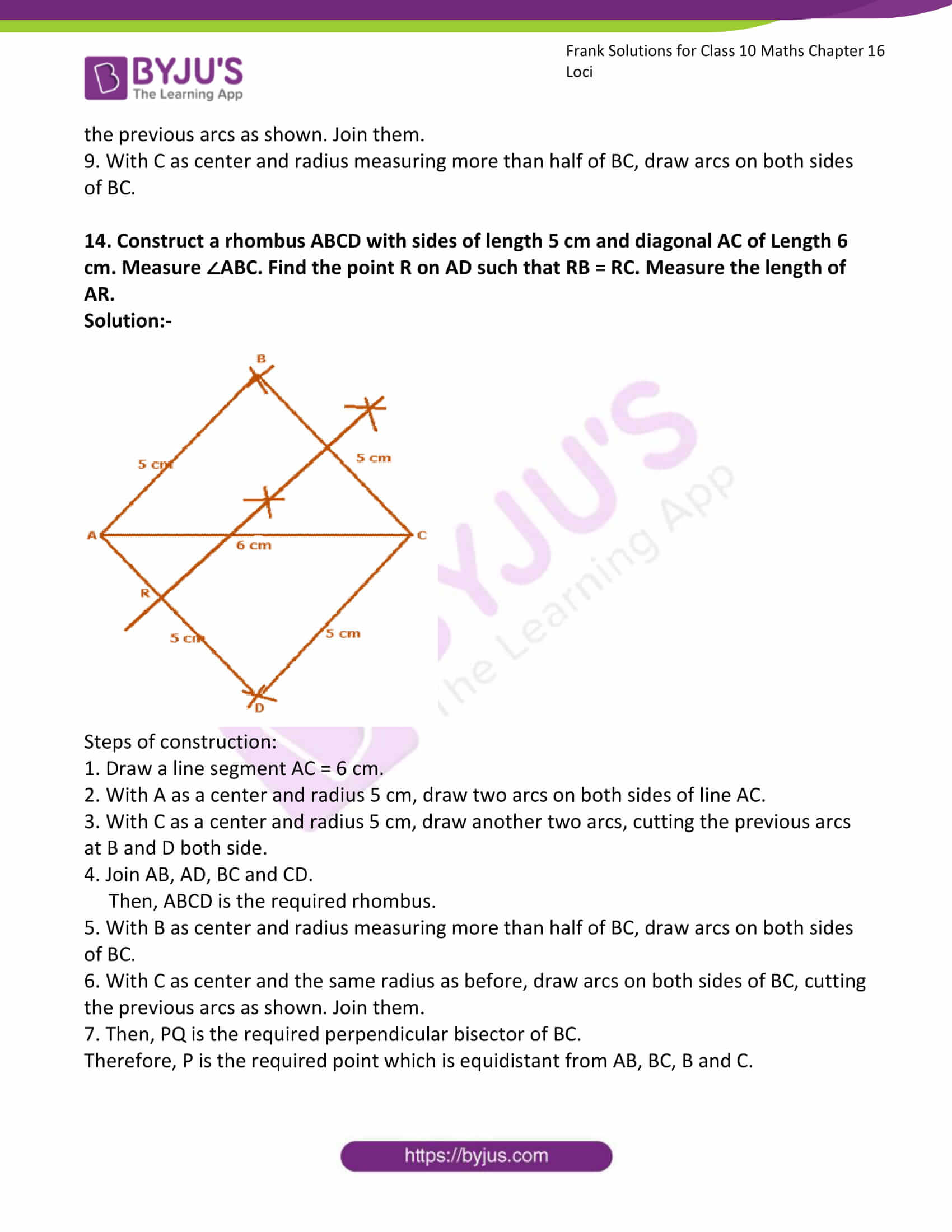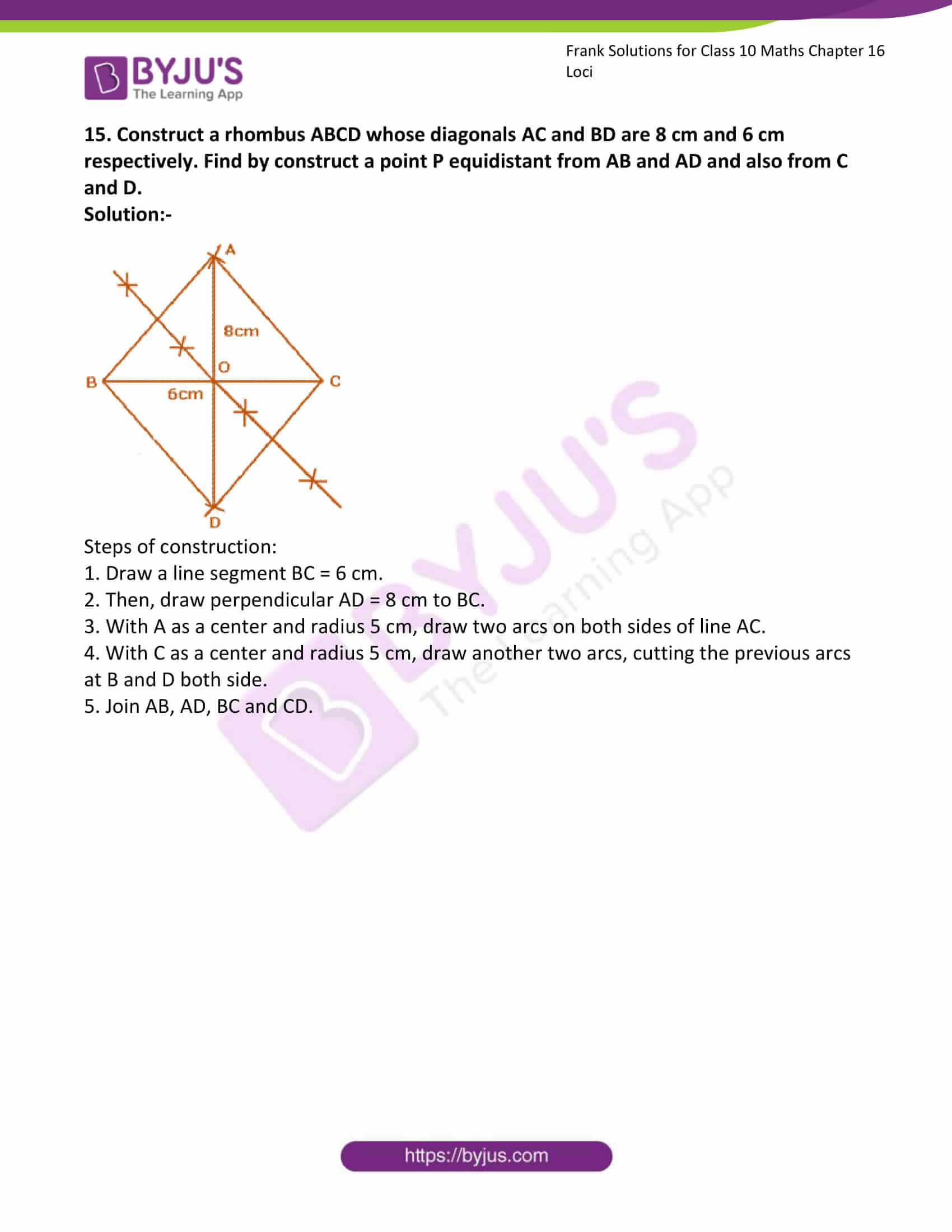### Access Answers to Frank Solutions for Class 10 Maths Chapter 16 Loci

1. Draw a straight line AB of 9 cm. Draw the locus of all points which are equidistant from A and B. Prove your statement.

Solution:-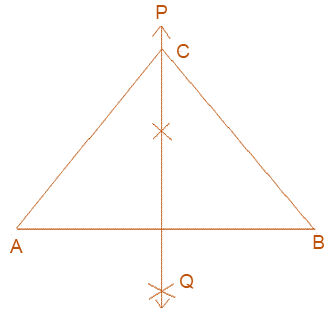Steps to construction:

(1) Draw a line segment AB of 9 cm

(2) Then draw perpendicular bisector PQ of AB. PQ is the required locus.

Proof:

(a) Take any point on PQ i.e. C.

(b) Now, join CA and CB.

Since, C lies on the perpendicular bisector of line AB.

So, C is equidistant from A and B.

Therefore, CA = CB

Hence, it is proved that perpendicular bisector of AB is the locus of all points which are equidistant from A and B.

2. A and B are fixed points while P is a moving point, moving in a way that it is always equidistant from A and B. What is the locus of the path traced out by the point P?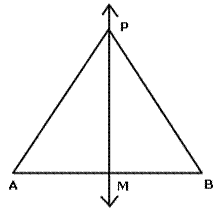Solution:-

The locus of the path traced out by the point P is equidistant from A and B is the perpendicular bisector of the line segment joining the two points.

3. A point moves such that its distance from a fixed line AB is always the same. What is the relation between AB and the path travelled by the point?

Solution:-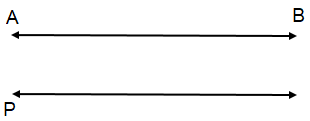A point moves such that its distance from a fixed straight line AB is the same as a pair of straight lines parallel to the given line, one on each side of it and at the given distance from it.

4. State the locus of a point moving so that its perpendicular distances from two given lines is always equal.

Solution:-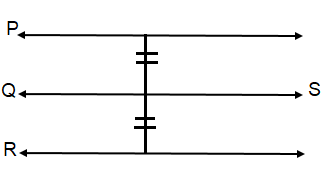The locus of a point moving so that its perpendicular distances from two given lines is always equal. A line QS parallel to given lines R and P.

5. AB is fixed line, state the locus of the point P such that ∠APB = 90o.

Solution:-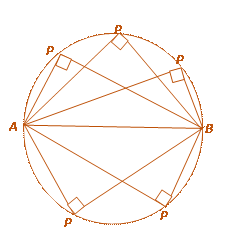The locus of the point P, such that ∠APB = 90o, with AB as diameter.

6. Draw and describe the locus in each of the following cases:

(a) The locus of points at a distance of 4 cm from a fixed line.

Solution:-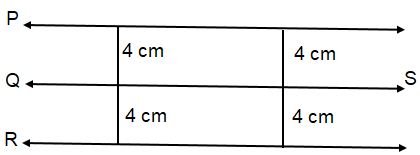The locus of points at a distance of 4 cm from a fixed line QS are lines R and P, which are parallel to QS.

(b) The locus of points inside a circle and equidistant from two fixed points on the circle.

Solution:-

The locus of points inside a circle are equidistant from fixed points on the circle will be the diameter which is the perpendicular bisector of the line joining the tow fixed points on the circle.(c) The locus of the mid – points of all parallel chords of a circle.

Solution:-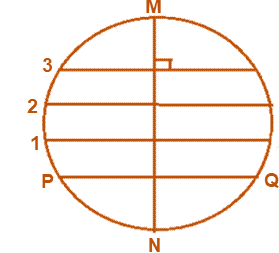The locus of the mid – points of the chords, which are parallel to a given chord, is the diameter perpendicular to the given chords.

(d) The locus of a point in rhombus ABCD, which is equidistant from AB and AD.

Solution:-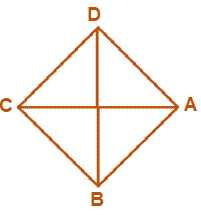7. Describe completely the locus of points in each of the following cases:

(a) Midpoint of radii of a circle.

Solution:-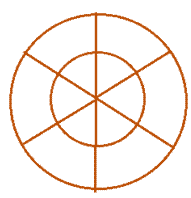(b) Centre of a ball, rolling along a straight line on a level floor.

Solution:-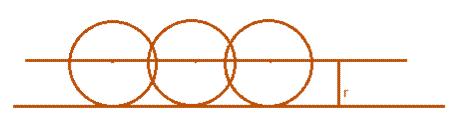(c) Point in a plane equidistant from a given line.

Solution:-(d) Centre of a circle of varying radius and touching the two arms of ∠ABC.

Solution:-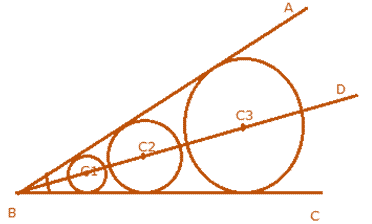(e) Centre of a circle of radius 2 cm and touching a fixed circle of radius 3 cm.

Solution:-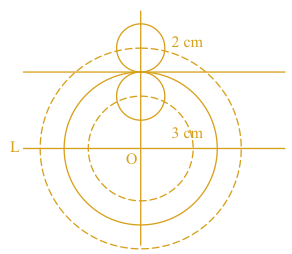8. Using only ruler and compasses, construct a triangle ABC, with AB = 5 cm, BC = 3.5 cm and AC = 4 cm. Mark a point P, which is equidistant from AB, BC and also from B and C. Measure the length of PB.

Solution:-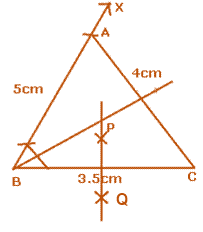Steps of construction:

1. Draw a line segment BC = 3.5 cm.

2. With B as a centre and radius 5 cm, draw an arc.

3. With C as a centre and radius 4 cm, draw another arc, cutting the previous arc at A.

4. Join AB and AC.

Then, ΔABC is the required triangle.

5. With B as centre and radius measuring more than half of BC, draw arcs on both sides of BC.

6. With C as centre and the same radius as before, draw arcs on both sides of BC, cutting the previous arcs at P and Q, as shown. Join PQ.

Then, PQ is the required perpendicular bisector of BC.

Therefore, P is the required point which is equidistant from AB, BC, B and C.

Length PB is 2.5 cm

9. Construct a triangle ABC, such that AB = 6 cm, BC = 7.3 cm and CA = 5.2 cm, locate a point which is equidistant from A, B and C.

Solution:-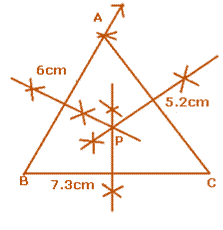Steps of construction:

1. Draw a line segment BC = 7.3 cm.

2. With B as a centre and radius 6 cm, draw an arc.

3. With C as a centre and radius 5.2 cm, draw another arc, cutting the previous arc at A.

4. Join AB and AC.

Then, ΔABC is the required triangle.

5. With B as centre and radius measuring more than half of BC, draw arcs on both sides of BC.

6. With C as centre and the same radius as before, draw arcs on both sides of BC, cutting the previous arcs as shown. Join them.

7. Again with B as centre and radius measuring more than half of BC, draw arcs on both sides of BC.

8. With A as centre and the same radius as before, draw arcs on both sides of BC, cutting the previous arcs as shown. Join them.

9. With C as centre and radius measuring more than half of BC, draw arcs on both sides of BC.

10. With A as centre and the same radius as before, draw arcs on both sides of BC, cutting the previous arcs as shown. Join them

So, in triangle ABC, P is the point of intersection of AB, AC and BC.

Therefore, PA =PB, PB = PC, PC = PA

Hence, P is the point which is equidistant from A, B and C.

10. Two straight roads AB and CD, cross each other at P at an angle of 75o. X is a stone on the road AB, 800m from P towards B, By taking an appropriate scale, draw a figure to locate the position of a pole, which is equidistant from P and X, and is also equidistant from the roads.

Solution:-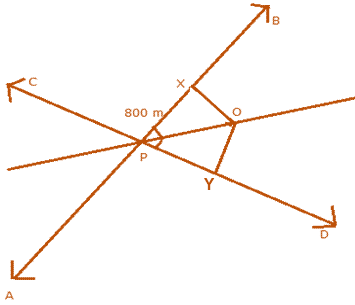Steps of construction:

As per the condition given in the question,

1. Draw two lines AB and CD crossing at an angle of 75o.

2. Then draw an angle bisector of ∠BPD

3. Now, draw a perpendicular from X on angle bisector meeting at O.

4. From point Y, PX = PY, draw a perpendicular on angle bisector meeting at O.

5. So, O is the point which equidistant from P, X and both the roads.

We know that, cos θ = hypotenuse/base

cos (75/2) = PO/PX

cos (37.5) = PO/800

0.980243 = PO/800

PO = 0.980243 × 800

PO = 784.19 m

11. Draw two intersecting lines to include an angle of 30o. Use ruler and compasses to locate points which are equidistant from these lines and also 2 cm away from their point of intersection. How many such points exist?

Solution:-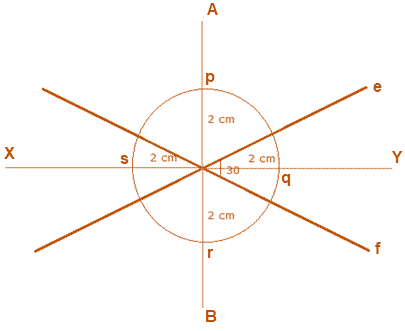As per the condition given in the question,

1. First, draw an angle bisector AB and XY of angles formed by the lines e and f.

2. Now, from centre draw a circle with radius 2 cm, which intersect the angle bisector at p, q, r and s, respectively.

Therefore, p, q, r and s are the required four points.

12. AB and CD are two intersecting lines. Find a point equidistant from AB and CD, and also at a distance of 1.8 cm from another given Line EF.

Solution:-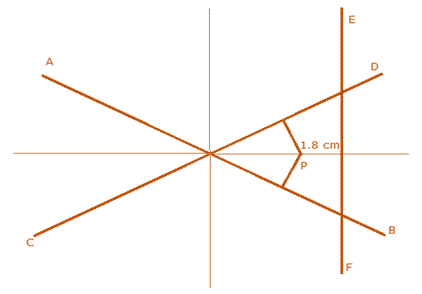As per the condition given in the question,

1. First draw an angle bisector AB and CD.

2. Then draw perpendicular from AB and CD on angle bisector i.e. P.

Therefore, P is the point equidistant from AB and CD, and also at a distance of 1.8 cm from another given Line EF.

13. Without using set squares or protractor, construct a quadrilateral ABCD in which ∠BAD = 45o, AD = AB = 6 cm, BC = 3.6 cm and CD = 5 cm. Locate the point P on BD, which is equidistant from BC and CD.

Solution:-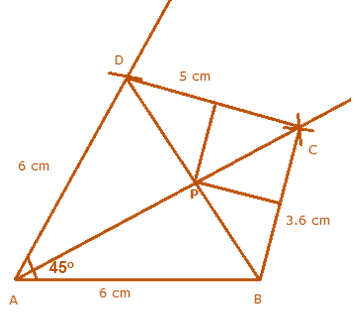Steps of construction:

1. Draw a line segment AB = 6 cm.

2. With B as a centre and radius 6 cm, draw an arc.

3. With C as a centre and radius 5.2 cm, draw another arc, cutting the previous arc at A.

4. Join AB and AC.

Then, ΔABC is the required triangle.

5. With B as centre and radius measuring more than half of BC, draw arcs on both sides of BC.

6. With C as centre and the same radius as before, draw arcs on both sides of BC, cutting the previous arcs as shown. Join them.

7. Again with B as centre and radius measuring more than half of BC, draw arcs on both sides of BC.

8. With A as centre and the same radius as before, draw arcs on both sides of BC, cutting the previous arcs as shown. Join them.

9. With C as centre and radius measuring more than half of BC, draw arcs on both sides of BC.

14. Construct a rhombus ABCD with sides of length 5 cm and diagonal AC of Length 6 cm. Measure ∠ABC. Find the point R on AD such that RB = RC. Measure the length of AR.

Solution:-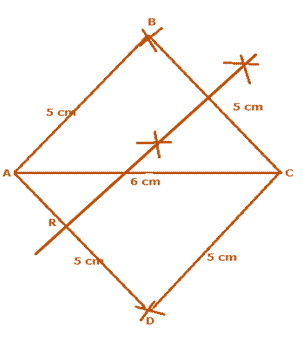Steps of construction:

1. Draw a line segment AC = 6 cm.

2. With A as a centre and radius 5 cm, draw two arcs on both sides of line AC.

3. With C as a centre and radius 5 cm, draw another two arcs, cutting the previous arcs at B and D both side.

4. Join AB, AD, BC and CD.

Then, ABCD is the required rhombus.

5. With B as centre and radius measuring more than half of BC, draw arcs on both sides of BC.

6. With C as centre and the same radius as before, draw arcs on both sides of BC, cutting the previous arcs as shown. Join them.

7. Then, PQ is the required perpendicular bisector of BC.

Therefore, P is the required point which is equidistant from AB, BC, B and C.

15. Construct a rhombus ABCD whose diagonals AC and BD are 8 cm and 6 cm, respectively. Find by construct a point P equidistant from AB and AD and also from C and D.

Solution:-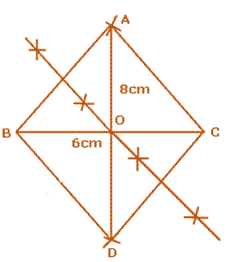Steps of construction:

1. Draw a line segment BC = 6 cm.

2. Then, draw perpendicular AD = 8 cm to BC.

3. With A as a centre and radius 5 cm, draw two arcs on both sides of line AC.

4. With C as a centre and radius 5 cm, draw another two arcs, cutting the previous arcs at B and D both side.

5. Join AB, AD, BC and CD.